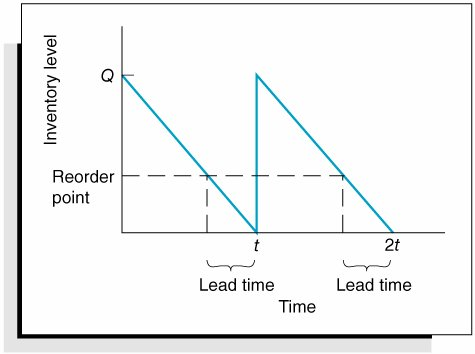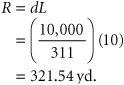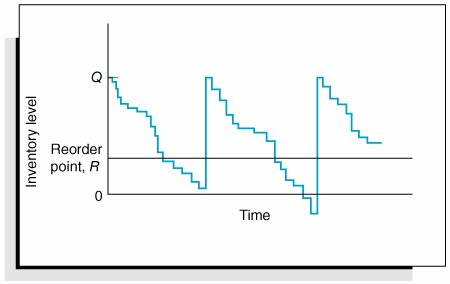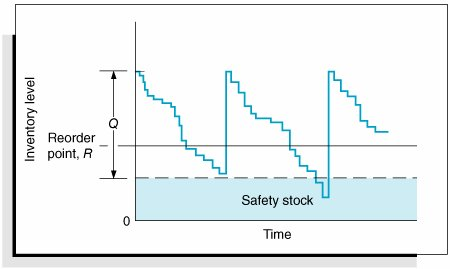# Reorder Point[Page 752 ( continued )]

In our presentation of the basic EOQ model in the previous section, we addressed one of the two primary questions related to inventory management: How much should be ordered? In this section we will discuss the other aspect of inventory management: When to order? The determinant of when to order in a continuous inventory system is the reorder point , the inventory level at which a new order is placed.

The concept of lead time is illustrated graphically in Figure 16.9. Notice that the order must be made prior to the time when the level of inventory falls to zero. Because demand is consuming the inventory while the order is being shipped, the order must be made while there is enough inventory in stock to meet demand during the lead-time period. This level of inventory is referred to as the reorder point and is so designated in Figure 16.9.

##### Figure 16.9. Reorder point and lead time[Page 753]

The reorder point for our basic EOQ model with constant demand and a constant lead time to receive an order is relatively straightforward. It is simply equal to the amount demanded during the lead-time period, computed using the following formula:

R = dL

where

d = demand rate per time period (i.e., daily)

L = lead time

Consider the I-75 Carpet Discount Store example described previously. The store is open 311 days per year. If annual demand is 10,000 yards of Super Shag carpet and the lead time to receive an order is 10 days, the reorder point for carpet is determined as follows :Thus, when the inventory level falls to approximately 321 yards of carpet, a new order is placed. Notice that the reorder point is not related to the optimal order quantity or any of the inventory costs.

#### Safety Stocks

In our previous example for determining the reorder point, an order is made when the inventory level reaches the reorder point. During the lead time, the remaining inventory in stock is depleted as a constant demand rate, such that the new order quantity arrives at exactly the same moment as the inventory level reaches zero in Figure 16.9. Realistically, however, demandand to a lesser extent lead timeis uncertain. The inventory level might be depleted at a slower or faster rate during lead time. This is depicted in Figure 16.10 for uncertain demand and a constant lead time.

##### Figure 16.10. Inventory model with uncertain demandNotice in the second order cycle that a stockout occurs when demand exceeds the available inventory in stock. As a hedge against stockouts when demand is uncertain, a safety (or buffer) stock of inventory is frequently added to the demand during lead time. The addition of a safety stock is shown in Figure 16.11.

[Page 754]
##### Figure 16.11. Inventory model with safety stockIntroduction to Management Science (10th Edition)
ISBN: 0136064361
EAN: 2147483647
Year: 2006
Pages: 358

Similar book on Amazon